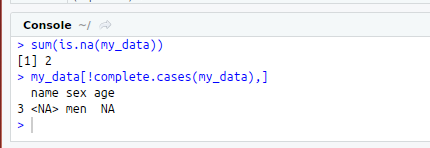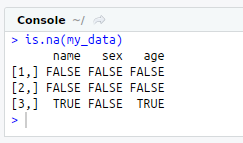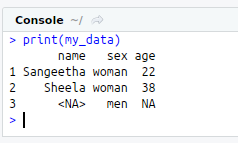• #5, First Floor, 4th Street Dr. Subbarayan Nagar Kodambakkam, Chennai-600 024 Landmark : Samiyar Madam
• pro@slogix.in
• +91- 81240 01111

### How to resolve Missing Values in R?

###### Description

To resolve the missing values using R programming.

###### Process
What is Missing Value?

In statistics, missing data, or missing values,occur when no data value is storedfor the variable in an observation.

#### Need of detecting Missing Data:

Missing data are a common occurrenceand can have a significant effecton the conclusionsthat can be drawnfrom the data.

Package and Function:

R Package : mice

To view the missing value in tabularform

To impute the missing values withmean/median/mode

R Function :na(x)-- To check whether there is any missingvalues in the given data frame ormatrix

R Function : sum(x)-- To find the number of missing values

R Function : cases(x)--To print a logical vector thatindicates complete and missing rows(i.e. rows without NA).

R Function : omit(x)-- To remove the columns having missingvalues

R Function : pattern(x)-- This function from MICE packageused to view the tabular form of missingValues

X -- Data Frame or Matrix

###### Sapmle Code

#Weibull Distribution

#dweibull
x<-seq(0,100,by=1)
y<-dweibull(x,shape = 3,scale=8)
plot(x,y,main=”dweibull plot”,col=”blue”)

#pweibull
y<-pweibull(x,shape=3,scale=10)
plot(x,y,main=”pweibull”,col=”red”)

#qweibull
y<-qweibull(p=0.4,shape = 2,scale = 2)
print(y)

#rweibull
x<-rweibull(n =20,shape = 4,scale = 4)
hist(x,col=275)

###### Screenshots